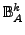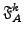# Mixed hyper-digital logic

online since July 2007

## Abstract

In previous texts (e.g. ), we defined bit table setsand their bit table algebras. Each one was fixed in the sense that carrier A and degree k were constants in the given context. We now ask for possibilities to define mixed algebras, in particular on

• the mixed carrier set Bk(A), which is the union of thefor all subsets S of A
• the mixed degree set B*(A), which is the union of the, for all degrees k
• the (general or mixed) bit table set B(A), which is the union of the, for all subsets S of A and all degrees k
The minimal condition we put on all these potential generalization will be, that they are proper expansions of all the fixed algebras they cover.

Similar to these versions of bit table algebras, we try to generalize the different "fixed" hyper-propositional formula algebrasby defining a mixed hyper-propositional formula algebra F(A).

## The text

... is under construction ...